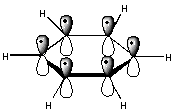# Why is phenyl carbocation unstable?

##### 1 Answer
Jun 27, 2015

The phenyl carbocation is unstable because of the high bond energy of the aromatic $\text{C-H}$ bond.

#### Explanation:

We can view the formation of a phenyl cation as

${\text{C"_6"H"_5"-H" → "C"_6"H"_5^+ +"H" + "e}}^{-}$

The $\text{C-H}$ bonds of benzene are ${\text{sp}}^{2}$ hybridized.

The high $\text{s}$ character means that the electrons are closer to the nucleus, so we must add more energy to remove these electrons and break the bond.

For example, it takes 423 kJ/mol to break the $\text{C-H}$ bond in ethane, but 470 kJ/mol in benzene.In addition, the vacant ${\text{sp}}^{2}$ orbital is in the plane of the ring.

It cannot overlap with the orbitals of the π system, so it cannot be stabilized by resonance.

The phenyl cation is a high-energy, unstable species.